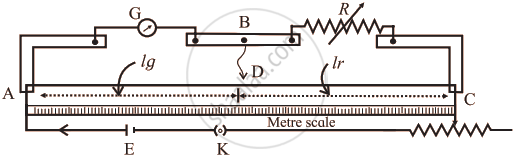# Describe Kelvin’s method to determine the resistance of the galvanometer by using a meter bridge. - Physics

Describe Kelvin’s method to determine the resistance of the galvanometer by using a meter bridge.

#### Solution

The galvanometer whose resistance (G) is to be determined is connected in one gap and a known resistance (R) in the other gap.Kelvin's Method

where,
G: Galvanometer,
R: Resistance box,
AC: Uniform resistance wire,
D: Balance point,
E: Cell,
K: Plug key,
Rh: Rheostat

The junction B  of the galvanometer and the resistance box is connected directly to a pencil jockey. A cell of emf E, a key (K) and a rheostat (Rh) are connected across AC.

Working:

1. A suitable resistance is taken in the resistance box. The current is sent round the circuit by closing the key. Without touching the jockey at any point of the wire, the deflection in the galvanometer is observed.
2. The rheostat is adjusted to get a suitable deflection Around (2/3)rd of range.
3. Now, the jockey is tapped at different points of the wire and a point of contact D for which, the galvanometer shows no change in the deflection, is found.
4. As the galvanometer shows the same deflection with or without contact between point B and D, these two points must be equipotential points.
5. The length of the bridge wire between the point D and the left end of the wire is measured. Let lg be the length of the segment of wire opposite to the galvanometer and lr be the length of the segment opposite to the resistance box.

Calculation:

Let RAD and RDC be the resistance of the two parts of the wire AD and DC respectively. Since the bridge is balanced

"G"/"R" = "R"_"AD"/"R"_"DC"

therefore "R"_"AD"/"R"_"DC" = l_"g"/l_"r"

therefore "G"/"R" = l_"g"/l_"r"

therefore "G"/"R" = l_"g"/(100 - l_"g")    ...(lg + lr = 100 cm)

G = (l_"g"/(100 - l_"g"))R

Using this formula, the unknown resistance of the galvanometer can be calculated.

Concept: Galvanometer
Is there an error in this question or solution?
Chapter 9: Current Electricity - Exercises [Page 228]

#### APPEARS IN

Balbharati Physics 12th Standard HSC Maharashtra State Board
Chapter 9 Current Electricity
Exercises | Q 5 | Page 228
Share